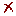Conversion

Power

 From ToMemo W W kW kW MW MW mW mW erg/s erg/s hp hp hp (metric) hp (metric) hp (boiler) hp (boiler) hp (electric) hp (electric) hp (U.K.) hp (U.K.) hp (water) hp (water) CV CV kcalIT/s kcalIT/s kcalth/s kcalth/s kcal/s kcal/s kgf.m/s kgf.m/s ft.lbf/s ft.lbf/s ft.lbf/min ft.lbf/min ft.lbf/h ft.lbf/h BTUIT/s BTUIT/s BTUth/s BTUth/s BTU/s BTU/s BTUIT/h BTUIT/h BTUth/h BTUth/h BTU/h BTU/h ton (Refrig) ton (Refrig) poncelet poncelet VA VA KVA KVA Power Factor =      Phase Angle (Ø) = ° To Memory 01 Memory 02 Memory 03 Memory 04 Memory 05 Memory 06 Replacing Adding Subtracting Multiplying Dividing

### Configurations

Do not use the number format settings
Maximum of decimal places

Accuracy of significant figures

Decimal separator

Thousands separator

Number Notation

Exponent Notation

Notation example:
12345.6 ou 1.23456e+4

Impression Page Title (Optional)